Year 1 ObjectivesPlace ValuePre Tasks

(PitStops)

Main Tasks

Addition and Subtraction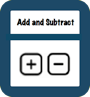Pre Tasks

(PitStops)

Main Tasks

Multiplication and Division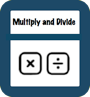Number - Fractions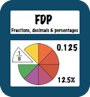Pre Tasks

(PitStops)

Main Tasks

Pre Tasks

(PitStops)

Main Tasks

- Recognise, find and name a half as one of two equal parts of an object, shape or quantity

- Recognise, find and name a quarter as one of four equal parts of an object, shape or quantity.1. -Count forwards and backwards to and across 100- Count, read and write numbers to 100 in numerals; count in multiples of twos, fives and tens

- Given a number, identify one more and one less

- Identify and represent numbers using objects and pictorial representations including the number line, and use the language of: equal to, more than, less than (fewer), most, least

- Read and write numbers from 1 to 20 in numerals and words.- Read, write and interpret mathematical statements involving addition (+), subtraction (–) and equals (=) signs

- Represent and use number bonds and related subtraction facts within 20

- Add and subtract one-digit and two-digit numbers to 20, including zero

- Solve one-step problems that involve addition and subtraction, using concrete objects and pictorial representations, and missing number problems such as 7 =  ___   –  9.- Solve one-step problems involving multiplication and division, by calculating the answer using concrete objects, pictorial representations and arrays with the support of the teacher.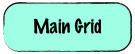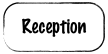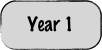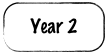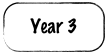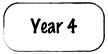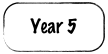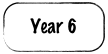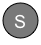Solutions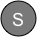StopsGap

StopsGap

StopsGap

StopsGap